|

# 狭小卫浴间的“微整形”技巧

让小的卫浴间看起来更大、更立体，墙体与地面的装修就显得尤为重要。在浴室空间设计的时候，材质、色彩都要逐一考虑到位，它们的选择会对浴室风格的形成起到决定性作用。狭小的卫浴间到底怎么设计才出彩呢，接下来，跟着编辑看看几位身披盖世经验战袍的业主家狭小卫浴 的“解码”吧。

part1、狭小卫浴解码之房型簿

小处不随便 卫浴不足5㎡怎么装

晓东：“我家是二手房，毕竟是老房子，搬进去前还是决定重新装修，不然心里别扭。卫浴间只有4.8㎡，虽小，干湿分离还是得注意，这关系到身体的健康。储蓄空间也尽可能多些。”

形状：狭长

面积：4.8平米

家庭结构：三口之家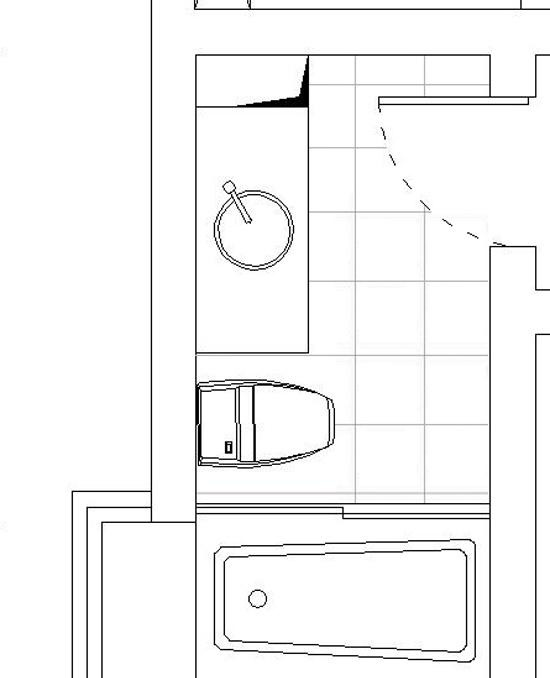卫生间平面图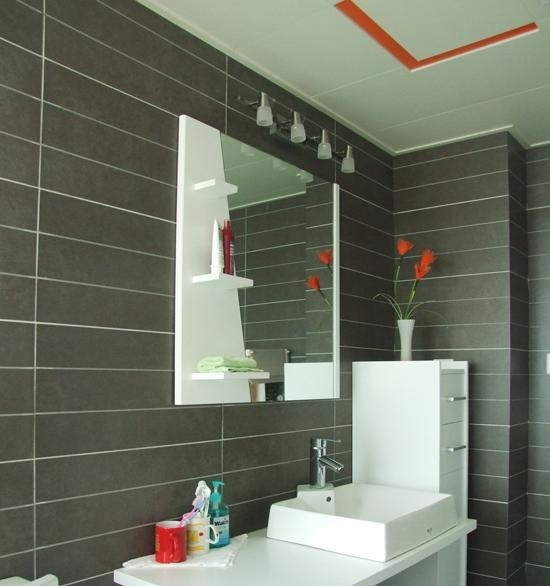可圈可点处

亮点：

1.镜子上有放置杂物的搁板，充分利用了空间，也很美观，辅助照明射灯也很实用。

2.台上盆美观大方，还容易清洁。

3.窄的收藏高柜，充分利用竖方向的空间。

败笔：

洗手台的设计过大，造成马桶的位置离淋浴房近了一些。

邢琳：“我家位于奥林匹克花园，浴室房型很正规，只是面积偏小。装修后布局基本合理，很符合生活规律。最大的缺陷是规划太过死板，没什么新意，一些空间没有利用完全。”

形状：方正

面积：4.5平米

家庭结构：小两口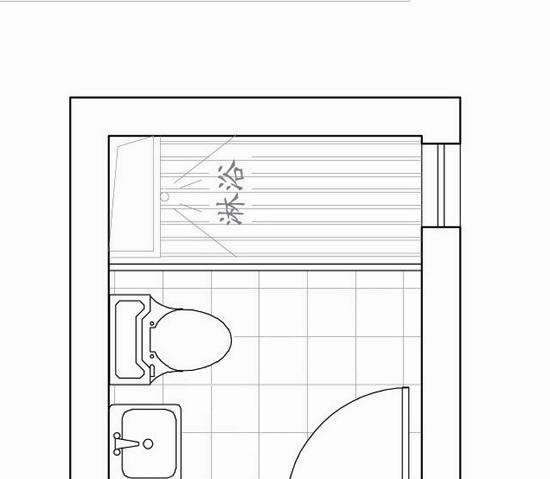卫生间平面图

可圈可点处亮点：

1.镜子和收纳柜结合在一起，省面积，一举两用。

2.干湿分离，卫生健康。

败笔：

1.可以选看不见电源的搁板，增加使用功能。

2.淋浴空间可以再小一点。

编辑点评

想让狭小的卫浴间释放几十倍的能量和空间，台盆、马桶的尺寸就要选合适的，或大或小都不好，一物多用也是小卫浴间节省空间的法宝。另外，在选材用料的时候也要注意，透光好的浴室总会显得很宽敞，小浴室得乖乖恪守这条法则。

part2、狭小卫浴解码之账本簿

精打细算装卫浴 5㎡账单明细揭晓

阿乐：“辛辛苦苦地赚钱图什么？不就是快快乐乐过日子。生活品质无论如何不能落下。我家卫浴面积不大，为符合我家的现代风格，整个空间以白色为主，在洁具选择上我很重视。”

估算：2600元/平米

面积：5㎡

主材：主材和辅材用的都是较好的品牌和材料，提高了整个卫浴间的品质，洁具的选择，花了不少的心思。

账本簿

墙地砖：诺贝尔 180元/㎡

吊顶：宝仕龙集成吊顶 3000元左右

洁具：科勒 3500元

台盆：科勒挂壁式 1400元

龙头：科勒 1500元

花洒：汉斯格雅 3600元

五金配件：1000元左右

科勒龙头佳佳：“卫浴间面积可以小，但各种功能还是得齐全，这关系到生活的舒适度。装修时，我们把主要重点放在了瓷砖、腰线、浴室柜的选择上，最后整个卫浴间的功能设计比较齐全。”

估算：3500元/平方米

面积：5㎡

主材：洁具和瓷砖都可以用一线品牌，比如伊奈、乐家、和成等，而瓷砖可以选用诺贝尔、马可波罗、东鹏等品牌。

账本簿

墙地砖：诺贝尔 350元/㎡

吊顶：防水石膏板 160元/㎡

洁具：伊奈+温水洗净便盖 8600元

台盆：科勒 4500元

龙头：科勒 2500元

花洒：高仪 6595元

五金配件：1500元

图伊奈座便器编辑点评

狭小卫生间在设计方面更要注意细节，空间层次，墙体腰线、花纹瓷砖、diy装饰，都是增加小浴室空间层次感的好方法。

part3、狭小卫浴解码之经验簿

5㎡当10㎡用 过来人收纳有话说

5平米，甚至5平米不足，这样的卫浴间在小户型大行其道的如今，越来越多地出现在我们的生活中。空间小没关系，最重要的是设计合理。若空间安排得当，小区域物尽其用，5㎡的面积大可以当做10㎡用。于是，收纳空间的充分利用，成为小卫浴间功能设置的关键。下面听听过来人是如何收纳的。

杨阿姨：把零碎物件都往墙上请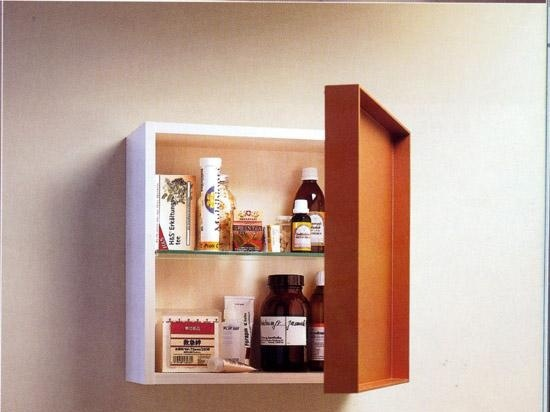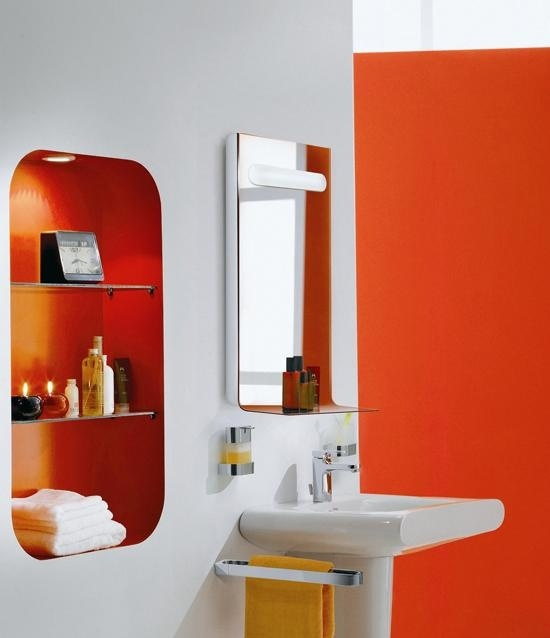美标产品

壁面是最好做收纳的地方，除了钉挂毛巾杆外，还有一些物品可以使用壁面空间收放，像是直接锁在墙面上的马桶刷或是悬吊式书架。毛巾、手纸、电吹风等等统统请上墙去。几块简单的玻璃板悬于墙壁上，就可以用来摆放化妆品。

莉莉：洗手台上下是宝地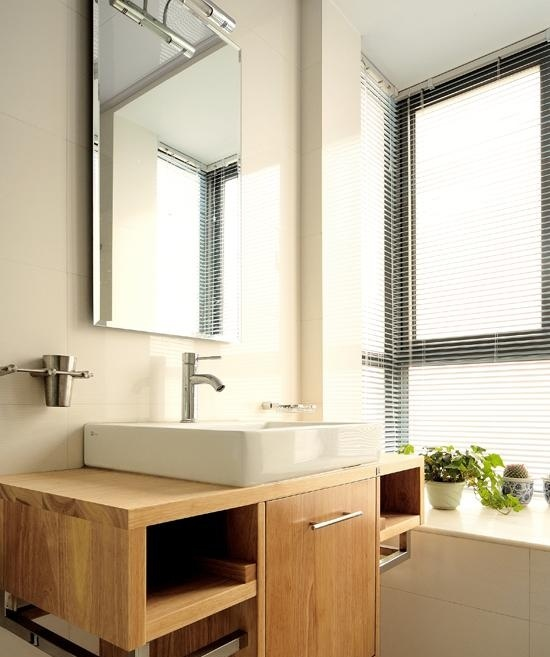吊柜加地柜加挂杆，即在洗面盆上方设置吊柜，下方安置地柜，旁边还可以安置挂杆，这样的方式能充分地利用洗手台四周的墙面；在墙壁里挖凹槽，将洗手台背后的墙面做出一个凹槽造型，把洗涤物品轻松搁在墙内，不占用卫浴空间自身的面积，还能使单调的墙面富于变化；内嵌台面加收纳盒，也就是将洗手台上方设计出一个台面，上面可放置收纳盒，把所用物品尽收其中，保持台面的整洁。

linda：窗台既要不遮光又要能储物连君曼设计

在窗户下设置一个小窗台就成了非常理想的收纳平台。但这个平台的收纳要注意不要遮光，不要杂乱。通常摆一盆小盆景，可以增添卫浴情趣，而卫浴中常用到的香熏也可以放在这里，窗户旁边通风利于挥发气味。

lina：放个小架子装着沐浴鞋

我们通常会在户门口设置鞋柜将淋浴时穿的鞋与日常鞋一起收纳，但这样做既很不方便，而且洗完澡后鞋总是滴水，不能及时摆放在鞋架上。不如在卫浴间入口区设置一个只能放两三双鞋的小鞋架，进出方便，又不占用很多空间。

许诺：坐便区四周能利用

杂志，还有卫生纸、清洁用品等的收纳是需要考虑的主要问题。可以在坐便器背后制作一个进深较浅、高度较高的收纳架子，用来放置杂志、清洁用品等。也可以制作一面假墙，将坐便器的水箱隐藏其中，再在假墙上挖凹槽，同样可以收纳很多杂物，也起到展示的功能。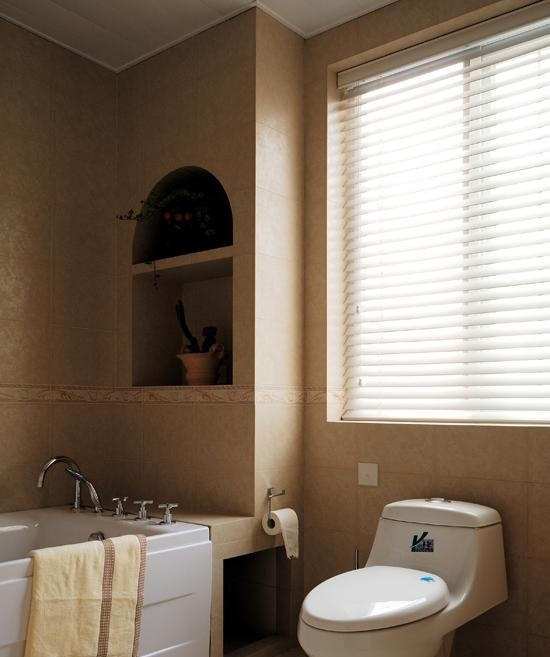连君曼设计

编辑点评

卫浴间里的零碎物件非常多，毛巾、卫生纸、护肤品、肥皂……形形色色，还都是小物件，一旦摆放不科学，就会使空间显得特别凌乱，所以它们都需要有个地方来安置。收纳柜想必是最不可少的收纳器皿，可以一股脑将小物件全部装上。所以，建议业主可以考虑收纳柜的购买。

part4、狭小卫浴解码之设备簿

卫浴洁具之“虾兵蟹将”少不了

空间设计全面部署妥当，便到了排兵布阵的时刻了。卫浴间的合理规划，能将功能区科学分配，而洁具的挑选自然也至关重要。选择恰当，房型上的缺点都能规避。市面上的卫浴品牌众多，多去市场逛逛，一定会有意外收获。下面就向大家推荐几款适合小卫浴间的洁具。

设备流要求一：

长相简洁，才有宽阔的视觉效果

现在由于越来越多小空间卫生间的出现，很多的卫浴产品为了迎合这样的趋势，而在设计上做出了一定的调整。很多线条简单，型号偏小的产品应运而生，让小空间在视觉上变得更大！充满刚性的直线条和代表柔和的曲线，配上简单的白色组合，外形精致，具有现代感，呈现出各式各样的时尚潮流感。品牌：乐家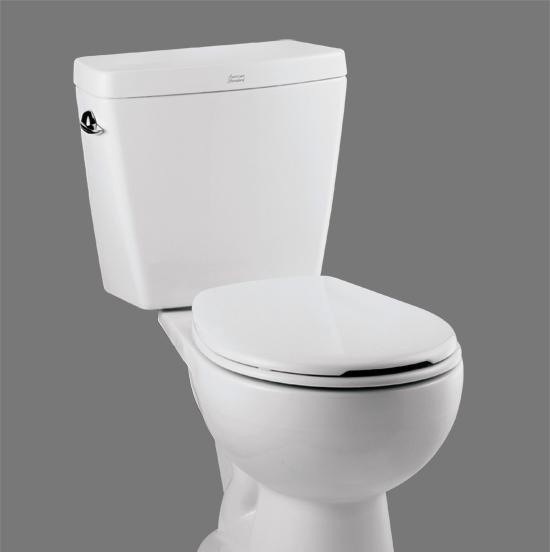品牌：美标 新埃高ros-a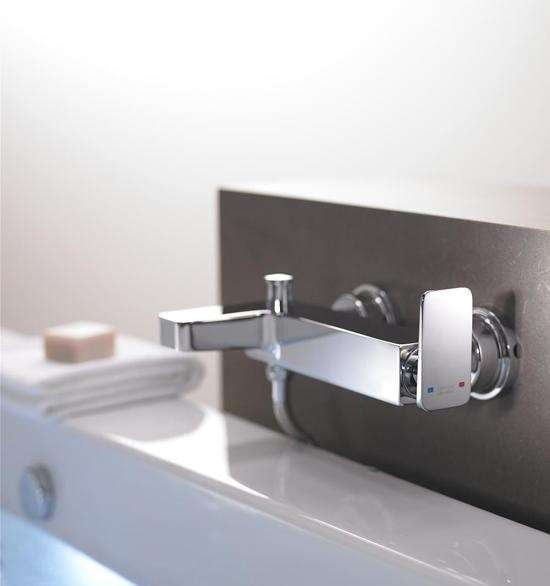品牌：美标 美康系列

设备流要求二：

体型灵巧，调遣才方便

小卫生间除了满足基本功能外，一般不应该设计过多的装饰品，而作为产品的选择，也要懂得巧妙使用。清扫空间最难的就是边角打扫，因为那是灰尘最容易去的地方，加上一些设备摆放，最容易积攒脏物，所以应该选用一些灵巧的设备。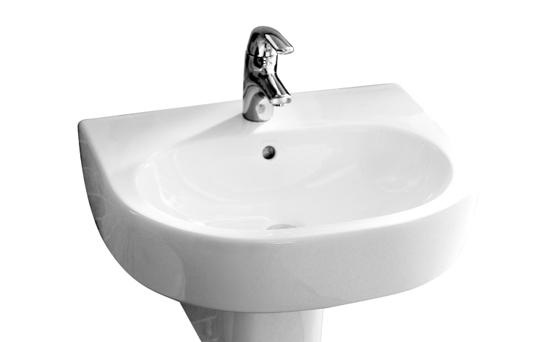科勒凯迪半柱式脸盆（不含龙头）

型号：k-17652t

规格：600×485mm

参考价：1140元/套

推荐理由：极富套间设计柱式脸盆感，优雅线条注重细节，充分节省卫浴空间。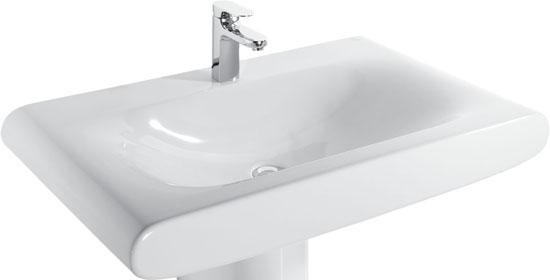美标美漫特半柱盆65cm

型号：cp-f088

规格：l650*w515*h425mm

参考价：1710元/套

推荐理由：采用了独特的内置式溢水系统idealflow；面盆的表面无溢水孔，更加美观，更具整体感，杜绝卫生死角与异味，更加易于清洁，更加健康；半入式设计，方便安装，节省空间。科勒飘瑞诗挂墙式橱柜

型号：k-3080t

规格：610*572*406mm

参考价：5085元/套

推荐理由：线条简洁，设计风格典雅，搭配飘瑞诗脸盆、台面及镜柜，和谐现代。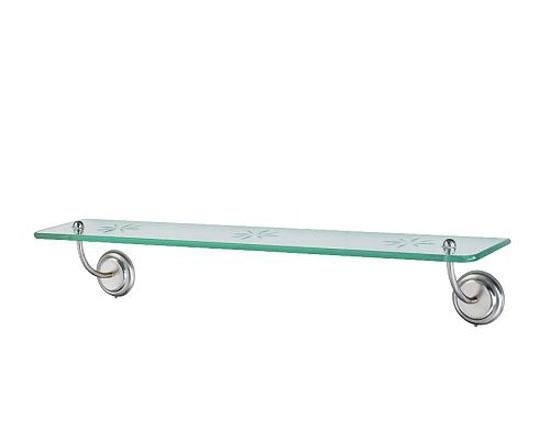宜家利霍蒙

参考价：79元/件

推荐理由：实惠的价格，方便的安装性。

设备流要求三：

综合素质，旮旯犄角都有大用处

小卫生间空间本来就已经十分捉襟见肘，在设计时要想做出大空间的效果，第一点就要充分利用卫生间的每一个角落。这就需要在选择洁具时，考虑到洁具的安装是否不同方位都能安装，或者安装起来并不费空间。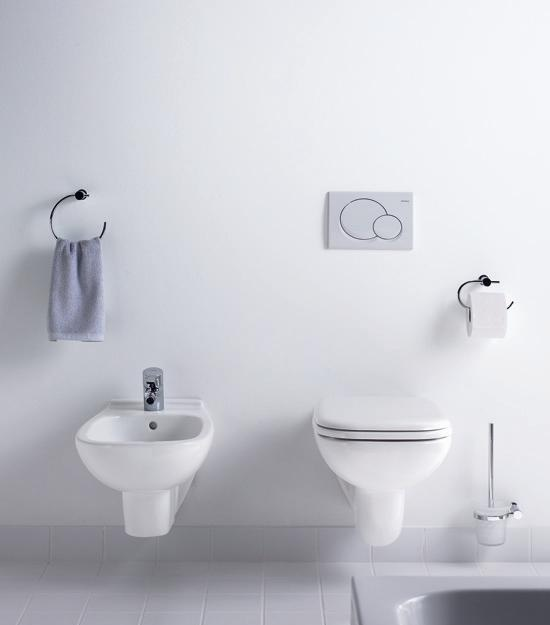品牌：杜拉维特d-code_009品牌：杜拉维特fogo_003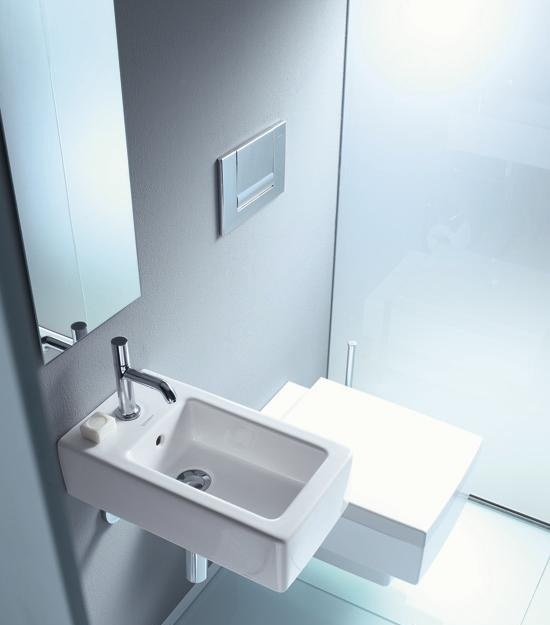品牌：杜拉维特fogo_004

编辑留言

有了完美的方案布局，也听了过来人的经验借鉴，解决狭小卫浴间造成困惑便是分分钟的事情了。只要方案合理、洁具配置科学，烦恼就总会烟消云散。

本文经《装修情报》授权独家发布，未经授权严禁在任何渠道平台以任何形式转载！

`声明：本文由入驻焦点开放平台的作者撰写，除焦点官方账号外，观点仅代表作者本人，不代表焦点立场错误信息举报电话： 400-099-0099，邮箱：jubao@vip.sohu.com，或点此进行意见反馈，或点此进行举报投诉。`A B C D E F G H J K L M N P Q R S T W X Y Z
A - B - C - D - E
• A
• 鞍山
• 安庆
• 安阳
• 安顺
• 安康
• 澳门
• B
• 北京
• 保定
• 包头
• 巴彦淖尔
• 本溪
• 蚌埠
• 亳州
• 滨州
• 北海
• 百色
• 巴中
• 毕节
• 保山
• 宝鸡
• 白银
• 巴州
• C
• 承德
• 沧州
• 长治
• 赤峰
• 朝阳
• 长春
• 常州
• 滁州
• 池州
• 长沙
• 常德
• 郴州
• 潮州
• 崇左
• 重庆
• 成都
• 楚雄
• 昌都
• 慈溪
• 常熟
• D
• 大同
• 大连
• 丹东
• 大庆
• 东营
• 德州
• 东莞
• 德阳
• 达州
• 大理
• 德宏
• 定西
• 儋州
• 东平
• E
• 鄂尔多斯
• 鄂州
• 恩施
F - G - H - I - J
• F
• 抚顺
• 阜新
• 阜阳
• 福州
• 抚州
• 佛山
• 防城港
• G
• 赣州
• 广州
• 桂林
• 贵港
• 广元
• 广安
• 贵阳
• 固原
• H
• 邯郸
• 衡水
• 呼和浩特
• 呼伦贝尔
• 葫芦岛
• 哈尔滨
• 黑河
• 淮安
• 杭州
• 湖州
• 合肥
• 淮南
• 淮北
• 黄山
• 菏泽
• 鹤壁
• 黄石
• 黄冈
• 衡阳
• 怀化
• 惠州
• 河源
• 贺州
• 河池
• 海口
• 红河
• 汉中
• 海东
• 怀来
• I
• J
• 晋中
• 锦州
• 吉林
• 鸡西
• 佳木斯
• 嘉兴
• 金华
• 景德镇
• 九江
• 吉安
• 济南
• 济宁
• 焦作
• 荆门
• 荆州
• 江门
• 揭阳
• 金昌
• 酒泉
• 嘉峪关
K - L - M - N - P
• K
• 开封
• 昆明
• 昆山
• L
• 廊坊
• 临汾
• 辽阳
• 连云港
• 丽水
• 六安
• 龙岩
• 莱芜
• 临沂
• 聊城
• 洛阳
• 漯河
• 娄底
• 柳州
• 来宾
• 泸州
• 乐山
• 六盘水
• 丽江
• 临沧
• 拉萨
• 林芝
• 兰州
• 陇南
• M
• 牡丹江
• 马鞍山
• 茂名
• 梅州
• 绵阳
• 眉山
• N
• 南京
• 南通
• 宁波
• 南平
• 宁德
• 南昌
• 南阳
• 南宁
• 内江
• 南充
• P
• 盘锦
• 莆田
• 平顶山
• 濮阳
• 攀枝花
• 普洱
• 平凉
Q - R - S - T - W
• Q
• 秦皇岛
• 齐齐哈尔
• 衢州
• 泉州
• 青岛
• 清远
• 钦州
• 黔南
• 曲靖
• 庆阳
• R
• 日照
• 日喀则
• S
• 石家庄
• 沈阳
• 双鸭山
• 绥化
• 上海
• 苏州
• 宿迁
• 绍兴
• 宿州
• 三明
• 上饶
• 三门峡
• 商丘
• 十堰
• 随州
• 邵阳
• 韶关
• 深圳
• 汕头
• 汕尾
• 三亚
• 三沙
• 遂宁
• 山南
• 商洛
• 石嘴山
• T
• 天津
• 唐山
• 太原
• 通辽
• 铁岭
• 泰州
• 台州
• 铜陵
• 泰安
• 铜仁
• 铜川
• 天水
• 天门
• W
• 乌海
• 乌兰察布
• 无锡
• 温州
• 芜湖
• 潍坊
• 威海
• 武汉
• 梧州
• 渭南
• 武威
• 吴忠
• 乌鲁木齐
X - Y - Z
• X
• 邢台
• 徐州
• 宣城
• 厦门
• 新乡
• 许昌
• 信阳
• 襄阳
• 孝感
• 咸宁
• 湘潭
• 湘西
• 西双版纳
• 西安
• 咸阳
• 西宁
• 仙桃
• 西昌
• Y
• 运城
• 营口
• 盐城
• 扬州
• 鹰潭
• 宜春
• 烟台
• 宜昌
• 岳阳
• 益阳
• 永州
• 阳江
• 云浮
• 玉林
• 宜宾
• 雅安
• 玉溪
• 延安
• 榆林
• 银川
• Z
• 张家口
• 镇江
• 舟山
• 漳州
• 淄博
• 枣庄
• 郑州
• 周口
• 驻马店
• 株洲
• 张家界
• 珠海
• 湛江
• 肇庆
• 中山
• 自贡
• 资阳
• 遵义
• 昭通
• 张掖
• 中卫

1室1厅1厨1卫1阳台

1
2
3
4
5

0
1
2

1

1

0
1
2
3报名成功，资料已提交审核A B C D E F G H J K L M N P Q R S T W X Y Z
A - B - C - D - E
• A
• 鞍山
• 安庆
• 安阳
• 安顺
• 安康
• 澳门
• B
• 北京
• 保定
• 包头
• 巴彦淖尔
• 本溪
• 蚌埠
• 亳州
• 滨州
• 北海
• 百色
• 巴中
• 毕节
• 保山
• 宝鸡
• 白银
• 巴州
• C
• 承德
• 沧州
• 长治
• 赤峰
• 朝阳
• 长春
• 常州
• 滁州
• 池州
• 长沙
• 常德
• 郴州
• 潮州
• 崇左
• 重庆
• 成都
• 楚雄
• 昌都
• 慈溪
• 常熟
• D
• 大同
• 大连
• 丹东
• 大庆
• 东营
• 德州
• 东莞
• 德阳
• 达州
• 大理
• 德宏
• 定西
• 儋州
• 东平
• E
• 鄂尔多斯
• 鄂州
• 恩施
F - G - H - I - J
• F
• 抚顺
• 阜新
• 阜阳
• 福州
• 抚州
• 佛山
• 防城港
• G
• 赣州
• 广州
• 桂林
• 贵港
• 广元
• 广安
• 贵阳
• 固原
• H
• 邯郸
• 衡水
• 呼和浩特
• 呼伦贝尔
• 葫芦岛
• 哈尔滨
• 黑河
• 淮安
• 杭州
• 湖州
• 合肥
• 淮南
• 淮北
• 黄山
• 菏泽
• 鹤壁
• 黄石
• 黄冈
• 衡阳
• 怀化
• 惠州
• 河源
• 贺州
• 河池
• 海口
• 红河
• 汉中
• 海东
• 怀来
• I
• J
• 晋中
• 锦州
• 吉林
• 鸡西
• 佳木斯
• 嘉兴
• 金华
• 景德镇
• 九江
• 吉安
• 济南
• 济宁
• 焦作
• 荆门
• 荆州
• 江门
• 揭阳
• 金昌
• 酒泉
• 嘉峪关
K - L - M - N - P
• K
• 开封
• 昆明
• 昆山
• L
• 廊坊
• 临汾
• 辽阳
• 连云港
• 丽水
• 六安
• 龙岩
• 莱芜
• 临沂
• 聊城
• 洛阳
• 漯河
• 娄底
• 柳州
• 来宾
• 泸州
• 乐山
• 六盘水
• 丽江
• 临沧
• 拉萨
• 林芝
• 兰州
• 陇南
• M
• 牡丹江
• 马鞍山
• 茂名
• 梅州
• 绵阳
• 眉山
• N
• 南京
• 南通
• 宁波
• 南平
• 宁德
• 南昌
• 南阳
• 南宁
• 内江
• 南充
• P
• 盘锦
• 莆田
• 平顶山
• 濮阳
• 攀枝花
• 普洱
• 平凉
Q - R - S - T - W
• Q
• 秦皇岛
• 齐齐哈尔
• 衢州
• 泉州
• 青岛
• 清远
• 钦州
• 黔南
• 曲靖
• 庆阳
• R
• 日照
• 日喀则
• S
• 石家庄
• 沈阳
• 双鸭山
• 绥化
• 上海
• 苏州
• 宿迁
• 绍兴
• 宿州
• 三明
• 上饶
• 三门峡
• 商丘
• 十堰
• 随州
• 邵阳
• 韶关
• 深圳
• 汕头
• 汕尾
• 三亚
• 三沙
• 遂宁
• 山南
• 商洛
• 石嘴山
• T
• 天津
• 唐山
• 太原
• 通辽
• 铁岭
• 泰州
• 台州
• 铜陵
• 泰安
• 铜仁
• 铜川
• 天水
• 天门
• W
• 乌海
• 乌兰察布
• 无锡
• 温州
• 芜湖
• 潍坊
• 威海
• 武汉
• 梧州
• 渭南
• 武威
• 吴忠
• 乌鲁木齐
X - Y - Z
• X
• 邢台
• 徐州
• 宣城
• 厦门
• 新乡
• 许昌
• 信阳
• 襄阳
• 孝感
• 咸宁
• 湘潭
• 湘西
• 西双版纳
• 西安
• 咸阳
• 西宁
• 仙桃
• 西昌
• Y
• 运城
• 营口
• 盐城
• 扬州
• 鹰潭
• 宜春
• 烟台
• 宜昌
• 岳阳
• 益阳
• 永州
• 阳江
• 云浮
• 玉林
• 宜宾
• 雅安
• 玉溪
• 延安
• 榆林
• 银川
• Z
• 张家口
• 镇江
• 舟山
• 漳州
• 淄博
• 枣庄
• 郑州
• 周口
• 驻马店
• 株洲
• 张家界
• 珠海
• 湛江
• 肇庆
• 中山
• 自贡
• 资阳
• 遵义
• 昭通
• 张掖
• 中卫• 手机• 分享
• 设计
免费设计
• 计算器
装修计算器
• 入驻
合作入驻
• 联系
联系我们
• 置顶
返回顶部